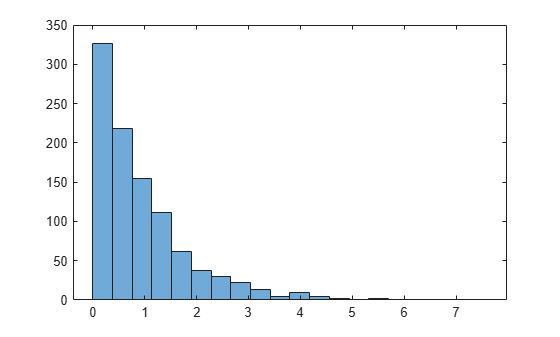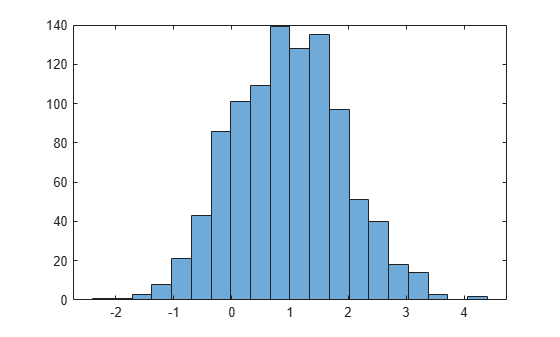Main Content

# Generate Random Numbers Using Uniform Distribution Inversion

This example shows how to generate random numbers using the uniform distribution inversion method. This is useful for distributions when it is possible to compute the inverse cumulative distribution function, but there is no support for sampling from the distribution directly.

### Step 1. Generate random numbers from the standard uniform distribution.

Use `rand` to generate 1000 random numbers from the uniform distribution on the interval (0,1).

```rng('default') % For reproducibility u = rand(1000,1);```

The inversion method relies on the principle that continuous cumulative distribution functions (cdfs) range uniformly over the open interval (0,1). If $u$ is a uniform random number on (0,1), then $x={F}^{-1}\left(u\right)$ generates a random number $x$ from any continuous distribution with the specified cdf `F`.

### Step 2. Generate random numbers from the Weibull distribution.

Use the inverse cumulative distribution function to generate the random numbers from a Weibull distribution with parameters `A = 1` and `B = 1` that correspond to the probabilities in `u`. Plot the results.

```x = wblinv(u,1,1); histogram(x,20);```The histogram shows that the random numbers generated using the Weibull inverse cdf function `wblinv` have a Weibull distribution.

### Step 3. Generate random numbers from the standard normal distribution.

The same values in `u` can generate random numbers from any distribution, for example the standard normal, by following the same procedure using the inverse cdf of the desired distribution.

```figure x_norm = norminv(u,1,1); histogram(x_norm,20)```The histogram shows that, by using the standard normal inverse cdf `norminv`, the random numbers generated from `u` now have a standard normal distribution.

Download ebook